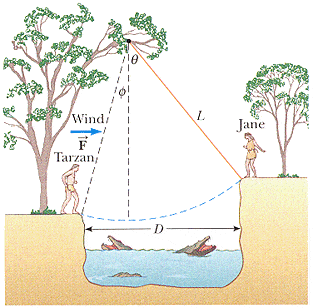# Simple harmonic motion rope swing problem

#### Ertosthnes

Jane wants to swing on a rope across a river. What minimum speed does she need to make it across, and once she's across, what minimum speed does she need to make it back?

Here's what's given:
mass = 47 kg
horizontal wind - call it F - (opposite to her swing) = 120 N
horizontal distance (D) = 50 m
rope length (L) = 40 m
theta = 50 degrees(hopefully you can see the image)

Here's how I started:

D = Lsin(theta) + Lsin(phi)

Plug in the values, and phi = 28.9 degrees

Then, Change in height = Lcos(phi) - Lcos(theta)
Plug in the values, change in height = 9.3 m

From there:

PE(o) + KE(o) + wind = PE(f) + KE(f)

mgh(0) + (1/2)mv(0)^2 - F(w)*D = mgh(f) + (1/2)mv(f)^2

and v(0) = 8.55 m/s

I got that part right. But, now how do I find the minimum velocity to go back? I tried switching the h(0) and h(f) in that last equation to go back, but it didn't work. What's wrong?

#### Hootenanny

Staff Emeritus
Gold Member
Have you considered that she is now swinging with the wind?

#### Ertosthnes

Yes, I did. Here's what happens:

mgh(0) + (1/2)mv(0)^2 + F(w)*D = mgh(f) + (1/2)mv(f)^2

46(9.8)(-9.3) + (1/2)(47)v(o)^2 + 120(50) = 0

and v(o)^2 = -76.92

But the fact that it's negative makes me think that it's wrong. Can I still take the square root of it?

Edit: Apparently I can. I just tried it again and got it right.

Last edited:
P

#### PSOA

##### Guest
But if you got a negative velocity, it means that the wind provides enough energy to Jane to reach that point, so that she doesn't need any initial velocity.

#### Ertosthnes

That's weird. Are you sure the negative doesn't just mean that Jane is swinging in the opposite direction? Is there something I should have done to make the velocity squared positive?

### The Physics Forums Way

We Value Quality
• Topics based on mainstream science
• Proper English grammar and spelling
We Value Civility
• Positive and compassionate attitudes
• Patience while debating
We Value Productivity
• Disciplined to remain on-topic
• Recognition of own weaknesses
• Solo and co-op problem solving